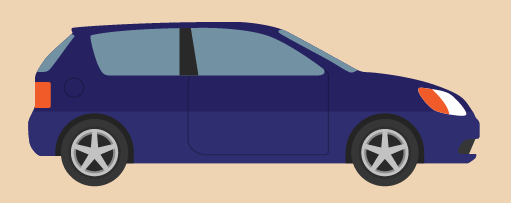Everyday maths for Health and Social Care and Education Support 1

Start this free course now. Just create an account and sign in. Enrol and complete the course for a free statement of participation or digital badge if available.

Free course

# 3.1 Fractions of amounts

Have a look at the following examples, which demonstrate how you would find the fraction of an amount.

## Example: Finding fractions

### Sarbjit is selling tickets for a charity concert at a school.Figure 13 A singer

of the funds raised will go to the school. Sarbjit makes £90 from selling tickets, so how much money will the school receive?

### Method

The basic rule for finding a unit fraction of an amount is to divide by the how many parts there are (the number on the bottom of the fraction) and multiply the result by the number at the top of the fraction:

of £90 is the same as £90 ÷ 3 = £30

The sum £30 × 1 = £30, so you would get £30 off.

### Survey

In a survey, of respondents said that they eat less than five portions of fruit and vegetables each day. If 800 people were surveyed, how many people eat less than five portions of fruit and vegetables each day?

### Method

Again, to find a fraction of an amount you need to divide by the number at the bottom of the fraction and then multiply that result by the number at the top of the fraction:

To answer this you need to first work out what of 800 people is.

of 800 = 800 ÷ 4 = 200

Then use the numerator (the top of the fraction) to work out how many of those unit fractions are needed:

of 800 = 3 × 200 = 600

So 600 people eat less than five portions of fruit and vegetables each day.

## Activity 10: Paying in instalmentsFigure 14 How much would a car cost?

Paul has recently qualified as a midwife and is going to be working out in the community. He needs his own car to travel to visit all of his patients.

Paul signs up to a 4-year PCP deal to purchase a car for £12,000. He needs to pay the money in stages:

1. of the money to be paid as an initial deposit.
2. of the money is to be paid over 4 years.
3. The remainder is to be paid at the end of the 4-year contract.

How much money will Paul need to pay at the end of the 4-year contract?

First you need to work out the initial deposit that Paul pays. So to work out of £12,000 you need to divide £12,000 by 5.

• 12,000 ÷ 5 = 2,400

So the deposit Paul has to pay is £2,400.

Then you need to work out of the £12,000 Paul needs to pay over the 4 years. Start by working out of £12,000. To do this you need to divide £12,000 by 3.

• 12,000 ÷ 3 = 4,000

So of £12,000 is:

• 4,000 × 2 = 8,000

So Paul will pay £8,000 over the 4 years.

Subtract these two amounts from the total cost to see how much Paul will have left to pay at the end of the contract.

• 12,000 – 2,400 – 8,000 = 1,600

Paul will need to pay £1,600 at the end of the 4-year contract.

## Summary

In this section you have learned how to:

• find equivalencies in fractions
• order and compare fractions
• find the fraction of an amount.

The skills listed above can be used when you are shopping and trying to get the best deal, or when you are splitting a cake or a pizza, say, into equal parts for a birthday celebration at a children’s care home.

It’s important to be able to compare fractions, decimals and percentages in real-life situations. You’ll be looking at percentages later, but first you can look at decimals.

FSM_SSH_1